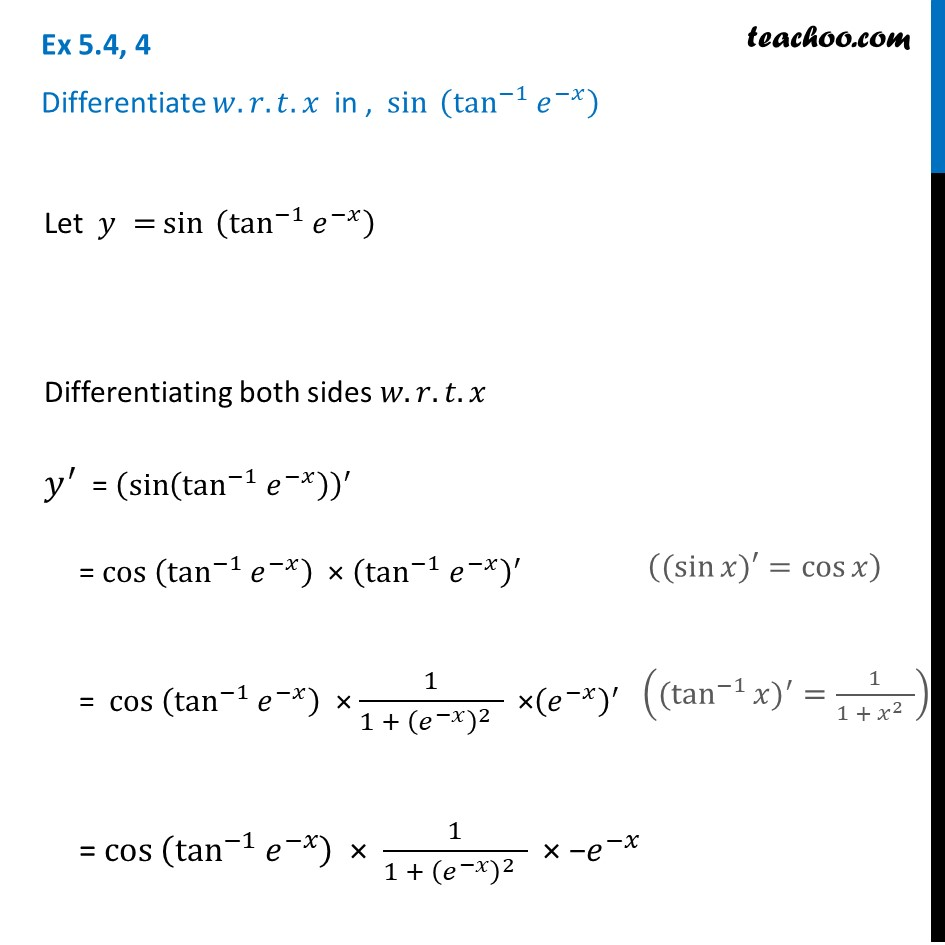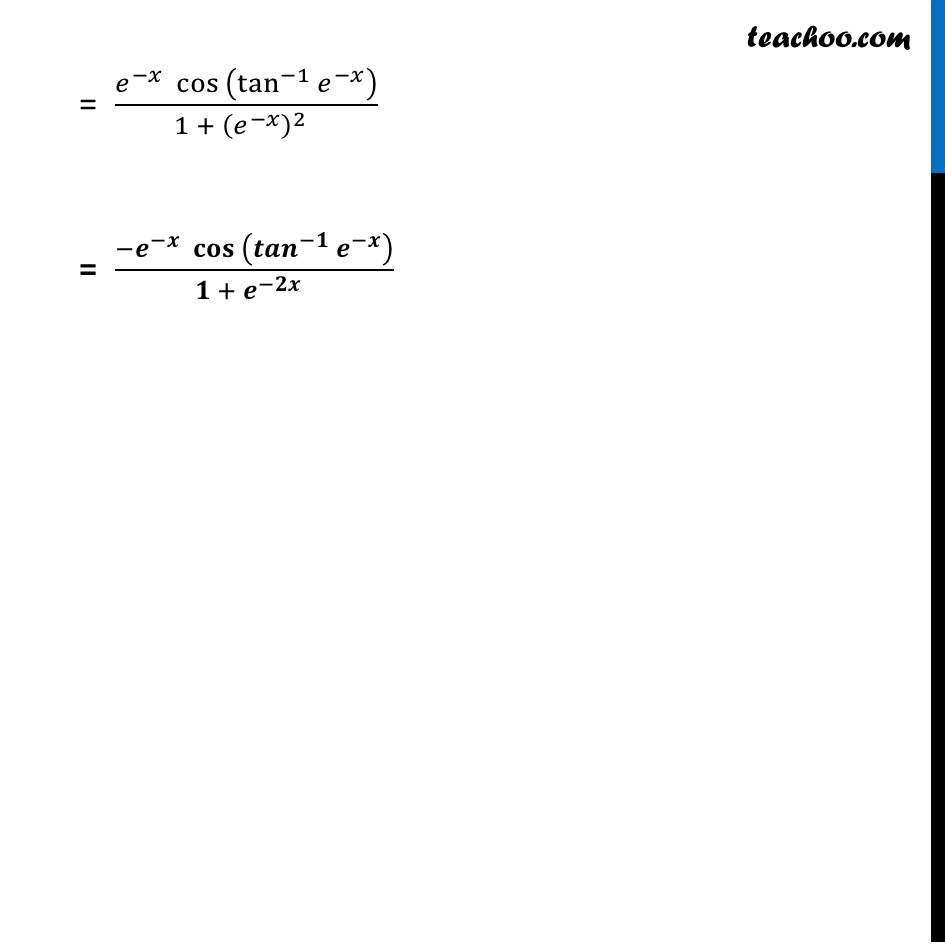Finding derivative of Exponential & logarithm functions

Chapter 5 Class 12 Continuity and Differentiability
Concept wiseIntroducing your new favourite teacher - Teachoo Black, at only ₹83 per month

### Transcript

Ex 5.4, 4 Differentiate 𝑤.𝑟.𝑡. 𝑥 in , sin⁡〖 (tan^(−1) 𝑒^(−𝑥) )〗Let 𝑦 = sin⁡〖 (tan^(−1) 𝑒^(−𝑥) )〗 Differentiating both sides 𝑤.𝑟.𝑡.𝑥 𝑦^′ = (sin⁡(tan^(−1) 𝑒^(−𝑥) ) )^′ = 〖cos 〗⁡(tan^(−1) 𝑒^(−𝑥) ) × (tan^(−1) 𝑒^(−𝑥) )^′ = 〖cos 〗⁡(tan^(−1) 𝑒^(−𝑥) ) × 1/(1 + (𝑒^(−𝑥) )^2 ) ×(𝑒^(−𝑥) )^′ = 〖cos 〗⁡(tan^(−1) 𝑒^(−𝑥) ) × 1/(1 + (𝑒^(−𝑥) )^2 ) × −𝑒^(−𝑥) = (𝑒^(−𝑥) 〖cos 〗⁡(tan^(−1) 𝑒^(−𝑥) ))/(1 + (𝑒^(−𝑥) )^2 ) = (−𝒆^(−𝒙) 〖𝐜𝐨𝐬 〗⁡(〖𝒕𝒂𝒏〗^(−𝟏) 𝒆^(−𝒙) ))/(𝟏 + 𝒆^(−𝟐𝒙) ) = (𝑒^(−𝑥) 〖cos 〗⁡(tan^(−1) 𝑒^(−𝑥) ))/(1 + (𝑒^(−𝑥) )^2 ) = (−𝒆^(−𝒙) 〖𝐜𝐨𝐬 〗⁡(〖𝒕𝒂𝒏〗^(−𝟏) 𝒆^(−𝒙) ))/(𝟏 + 𝒆^(−𝟐𝒙) ) = (𝑒^(−𝑥) 〖cos 〗⁡(tan^(−1) 𝑒^(−𝑥) ))/(1 + (𝑒^(−𝑥) )^2 ) = (−𝒆^(−𝒙) 〖𝐜𝐨𝐬 〗⁡(〖𝒕𝒂𝒏〗^(−𝟏) 𝒆^(−𝒙) ))/(𝟏 + 𝒆^(−𝟐𝒙) )